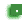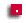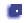## Random number programs

There are a whole mess of programs to do. Most are very short and quick. It's smartest to do the simple programs first (green then red then blue)

###Random1.java

Print 20 random integers that range from 1 to 200.

###Random2.java

Print 20 random doubles that range from 0.0 to 10.0

###Dice1.java

Print 20 random numbers that go from 1 to 6 (like a dice). In your program count how many 3s there are and print it out. Check this by looking at the 20 numbers.

###Dice2.java

Simulate rolling a dice 600 times.
Count up how many 1s, 2s, 3s, ...6s there are and print this out.
(Don't print out all 600 numbers!)
There should be approximately 100 of each number, right?

This is really easy to do using arrays: Make an array of size 7, then `numbers[r]++;`

###CloseNum.java

In a while loop, create two random numbers between 1 and 100. If they are within 4 of each other (e.g. 16 and 20, or 34 and 32), then exit.
Count how many times you have to loop to get two random numbers that are close to each other.

###Two67.java

Make random numbers between 1 and 100. How many numbers have to be generated before you get two 67s in a row?